# Overdetermined system

Overdetermined system

In mathematics, a system of linear equations is considered overdetermined if there are more equations than unknowns. The terminology can be described in terms of the concept of counting constraints. Each unknown can be seen as an available degree of freedom. Each equation introduced into the system can be viewed as a constraint that restricts one degree of freedom.

Therefore the critical case occurs when the number of equations and the number of independent variables are equal. For every degree of freedom, there exists a corresponding restraint. The overdetermined case occurs when the system has been overconstrained—that is, when the number of equations outnumbers the number of the unknowns.

## Systems of equations

### An example in two dimensions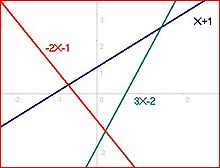#1 A system of three equations, three lines, three intersections, pairwise linearly independent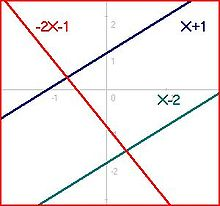#2 A system of three equations, three lines (two parallel), two intersections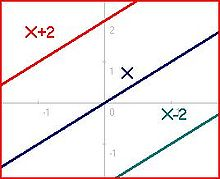#3 A system of three equations, three lines (three parallel), no intersections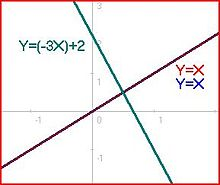#4 A system of three equations, three lines (two collinear), one intersection, one linearly dependent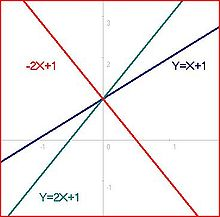#5 A system of three equations, three lines, one intersection, one linearly dependent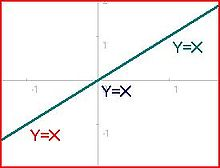#6 A system of three equations, three lines, infinite intersection, all linearly dependent

Consider the system of 3 equations and 2 unknowns (x1 and x2):

2x1 + x2 = − 1

− 3x1 + x2 = − 2

x1 + x2 = 1

Note: equations above correspond to picture #1 such that x1 = x and x2 = y in the Cartesian coordinate plane

There are three "solutions" to the system as can be determined from the graph's intersections, one for each pair of linear equations: between one and two (0.2, −1.4), between one and three (−2/3, 1/3), between two and three (1.5, 2.5). However there is no solution that satisfies all three simultaneously. Systems of this variety are deemed inconsistent.

The only case where the overdetermined system does in fact have a solution is demonstrated in pictures four, five, and six. These exceptions occur when the overdetermined set contains linearly dependent equations. Linear dependence means that the elements of a set can be described in terms of existing equations. For example, y = x + 1 and 2y = 2x + 2 are linearly dependent equations.

### Matrix form

Any system of linear equations can be written as a matrix equation. The previous system of equations can be written as follows:$\begin{bmatrix} 2 & 1 \\ -3 & 1 \\ -1 & 1 \\ \end{bmatrix} \begin{bmatrix} X_1 \\ X_2 \\ \end{bmatrix} = \begin{bmatrix} -1 \\ -2 \\ 1 \\ \end{bmatrix}$

Notice that the number of rows outnumber the number of columns. In linear algebra the concepts of row space, column space and null space are important for determining the properties of matrices. The informal discussion of constraints and degrees of freedom above relate directly to these more formal concepts.

## General cases

### Homogeneous case

The homogeneous case is always consistent (because there is a trivial, all-zero solution). There are two cases, in rough terms: there is just the trivial solution, or the trivial solution plus an infinite set of solutions, of nature determined by the number of linearly dependent equations.

Consider the system of linear equations: Li = 0 for 1 ≤ iM, and variables X1, X2, ..., XN, then X1 = X2 = ... = XN = 0 is always a solution. When M < N the system is underdetermined and there are always further solutions. In fact the dimension of the space of solutions is always at least NM.

For MN, there may be no solution other than all values being 0. There will be other solutions just when the system of equations has enough dependencies (linearly dependent elements), so that the number of effective constraints is less than the apparent number M; more precisely the system must reduce to at most N − 1 equations. All we can be sure about is that it will reduce to at most N.

### Inhomogeneous case

When studying systems of linear equations, Li=ci for 1 ≤ iM, in variables X1, X2, ..., XN the equations Li are sometimes linearly dependent. These dependent equations (often described in terms of vectors) lead to three possible cases for an overdetermined system.

• M equations* and N unknowns*, such that M > N and all M are linearly independent. This case yields no solution.
• M equations and N unknowns, such that M > N but all M are not linearly independent. There exist three possible sub-cases of this:
• M equations and N unknowns, such that M > N but all M are not linearly independent, but when the linearly dependent equations(D) are removed M - D > N. This case yields no solutions.
• M equations and N unknowns, such that M > N but all M are not linearly independent, but when the linearly dependent equations(D) are removed M - D = N. This case yields a single solution.
• M equations and N unknowns, such that M > N but all M are not linearly independent, but when the linearly dependent equations(D) are removed M - D < N. This case yields infinitely many solutions. Which can be described as F which is the entirety of the field in which the equations are operating.

Note:(*Equations and unknowns can correspond to the rows and columns of a matrix respectively)

• M equations and N unknowns, such that M < N and all M are linearly dependent. This case yields infinitely many solutions except in some cases where the solution is sparse (see Compressed Sensing)

The discussion is more convincing, perhaps, when translated into the geometric language of intersecting hyperplanes. The homogeneous case applies to hyperplanes through a given point, taken as origin of coordinates. The inhomogeneous case is for general hyperplanes, which may therefore exhibit parallelism or intersect. A sequence of hyperplanes H1, H2, ..., HM gives rise to intersections of the first k, which are expected to drop in dimension 1 each time. If M > N, the dimension of the ambient space, we expect the intersection to be empty, and this is precisely the overdetermined case.

## Approximate solutions

The method of least squares can be used to find an approximate solution to overdetermined systems. For the system Ax = b, the least squares formula can be written x = (A'A) − 1A'b with this formula an approximate solution is found.$\min_{x}\Vert Ax - b \Vert$

## In general use

The concept can also be applied to more general systems of equations, such as partial differential equations.

Wikimedia Foundation. 2010.

### Look at other dictionaries:

• overdetermined — adjective a) (Of a problem or question) which suffers so many constraints that it has no solution. b) (Of a system of linear equations) which has more equations than variables …   Wiktionary

• Global Positioning System — GPS redirects here. For other uses, see GPS (disambiguation). Geodesy Fundamentals …   Wikipedia

• Integrable system — In mathematics and physics, there are various distinct notions that are referred to under the name of integrable systems. In the general theory of differential systems, there is Frobenius integrability, which refers to overdetermined systems. In… …   Wikipedia

• Linear least squares — is an important computational problem, that arises primarily in applications when it is desired to fit a linear mathematical model to measurements obtained from experiments. The goals of linear least squares are to extract predictions from the… …   Wikipedia

• Linear least squares/Proposed — Linear least squares is an important computational problem, that arises primarily in applications when it is desired to fit a linear mathematical model to observations obtained from experiments. Mathematically, it can be stated as the problem of… …   Wikipedia

• Linear least squares (mathematics) — This article is about the mathematics that underlie curve fitting using linear least squares. For statistical regression analysis using least squares, see linear regression. For linear regression on a single variable, see simple linear regression …   Wikipedia

• Simultaneous equations — In mathematics simultaneous equations are a set of equations containing multiple variables. This set is often referred to as a system of equations. To solve simultaneous equations, the solver needs to use the provided equations to find the exact… …   Wikipedia

• Ambiguity — Sir John Tenniel s illustration of the Caterpillar for Lewis Carroll s Alice s Adventures in Wonderland is noted for its ambiguous central figure, whose head can be viewed as being a human male s face with a pointed nose and pointy chin or being… …   Wikipedia

• Least squares — The method of least squares is a standard approach to the approximate solution of overdetermined systems, i.e., sets of equations in which there are more equations than unknowns. Least squares means that the overall solution minimizes the sum of… …   Wikipedia

• Overdetermination — For the mathematics term, see Overdetermined system. Overdetermination, the idea that a single observed effect is determined by multiple causes at once (any one of which alone might be enough to account for the effect), was originally a key… …   Wikipedia